# An Improved Single Phase Self-balancing Switched Capacitor Based Step-up Nine Level Inverter

An Improved Single Phase Self-balancing Switched Capacitor Based Step-up Nine Level Inverter
Department of Electrical and Electronics Engineering, Vignan's Foundation for Science, Technology & Research, Vadlamudi, Guntur 522213, India

Department of Electrical and Electronics Engineering, Vignan's Lara Institute of Technology & Science, Vadlamudi, Guntur 522213, India

Corresponding Author Email:
sgb_eeep@vignan.ac.in
Page:
249-257
|
DOI:
https://doi.org/10.18280/jesa.530212
23 December 2019
|
Accepted:
10 February 2020
|
Published:
20 May 2020
| Citation

OPEN ACCESS

Abstract:

The improved single phase switched capacitor based nine level inverter is presented in this article. A low DC input voltage is transformed into AC and boost up to the high output voltage without any Boost converter, inductors, transformers. The self-balancing process is involved in the states of charging and discharging of the capacitors. The presented topology does not have any H-bridge configurations which result in the low Total Standing Voltage (TSV). The Phase Disposition Carrier based PWM (PDCPWM) control technique is applied to the presented nine level inverter. The conduction loss, switching loss, efficiency and capacitor ratings are analyzed mathematically. The comparative analysis of the number of semiconductor switches, Total Standing Voltage, Peak Inverse Voltage between the presented topology and existing topology is explained in detail. Finally, using MATLAB/SIMULINK the proposed nine level inverter is simulated to realize the performance of the presented topology.

Keywords:

Total Standing Voltage (TSV), Phase Disposition Carrier based PWM (PDCPWM), self-balancing, switched-capacitor, Peak Inverse Voltage (PIV)

1. Introduction

Nowadays, Multilevel Inverters (MI's) have become very popular in usage for the applications in renewable energy systems, industries, electric vehicles, HVDC transmission systems . The Multilevel Inverters have low dv/dt, high efficiency, improves the quality of output . The three classical topologies NPC [3, 4], FC [5, 6], CHB [7, 8] are widely using in many applications. For more levels of output, the CHB, NPC, and FC requires more gate driver circuits, semiconductor devices, and facing problems in balancing the capacitor voltages . In many industrial applications, the high output AC voltage is required from the low input DC voltage . For this purpose, the transformer or boost converter based topologies are preferred. But, these inductor or transformer topologies have high volumes of cores, require more filtering circuits which leads to expensive, complexity and bulky in size . The power electronics researchers and industrialists of all over the world are inventing the Transformer-less inverter topologies to obtain the more levels of DC-AC output voltage with less number of semiconductor devices, low Total Standing Voltage (TSV) and Peak Inverse Voltage (PIV) .

The switched diode-based cascaded H-Bridge Multilevel inverter of two-stage configuration with several numbers of DC sources has been proposed by Wang et al. . The modules of switched diode produce the number of levels in the first stage, the H-bridge inverter generates the polarity in the second stage with less number of switches. The switched capacitor based H-bridge Inverter with self-balancing capability is proposed by Hinago and Koizumi . For more levels of output voltage, the switches voltage stress is very high, leads to high TSV and PIV. The alternate connected switched DC sources  are placed in opposite polarities through the semiconductor switches with boosting capability that will produce an output voltage. For high levels of output, the number of DC sources is required which causes the high voltage stress between the semiconductor switches. The Packed U-Cells (PUC) inverter  has a single DC source, with self-balancing capacitors generates the high levels of the DC-AC output voltage. The system will become very complex, increases the voltage stress of switches for the high number of levels. The Switched capacitor boost inverter  has a single DC source, four capacitors with the self-balancing capability to produce a nine-level inverter. The requirement of capacitors is high for increasing the number of levels. The self-balanced based switched capacitor boost multilevel inverter  is shown in Figure 1, voltage stress of each switch is the same which is equal to input DC voltage, which results in low TSV and PIV. The requirement of semiconductor switches, gate drivers is more in this topology which leads to system bulky and expensive.

An improved switched-capacitor based nine-level inverter without H-bridge configuration is presented in this article. It has a single DC source, less number of semiconductor switches and capacitor compared to the earlier topologies. The self-balancing process is utilized for charging and discharging of the capacitors. The level shift PWM technique is preferred to this topology to provide gate pulses to the switches. The novelty in this topology is maintaining a low Total Standing Voltage and Peak Inverse voltage with less number of switches which reduces the losses and cost of the system. The performance of topology is analyzed by THD analysis with a modulation index of 0.9. Due to having boosting voltage ability, this inverter is offered to applications such as Renewable Energy Systems (RES), Electric Vehicles (EVs), Uninterruptible Power Supplies (UPS).

## 1.jpg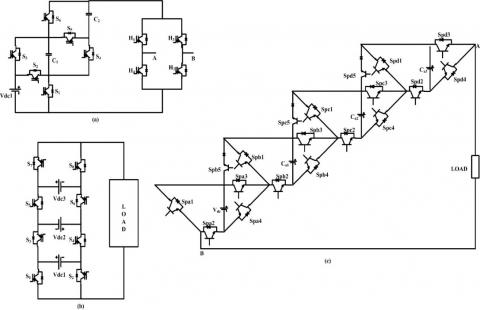Figure 1. Existing topologies (a) (b)  (c) 

2. Analysis of Improved Nine Level Inverter

## 2.jpgFigure 2. Improved switched-capacitor based nine-level inverter

The improved switched-capacitor based nine-level inverter is given in Figure 2. This topology requires a single DC source, twelve semiconductor switches, and two capacitors. The switches pair Sp1 & Sp2, Sp3 & Sp4, Sp5 & Sp6, Sp9 & Sp10 are operated complementary. For the charging and the discharging of capacitors, the self-balancing process is utilized. This topology will boost the voltage of 2Vin from the application of input voltage VDC with the nine-level output. The standing voltage of switches Sp1, Sp2, Sp3, Sp4, Sp5, Sp6, Sp9, Sp10, Sp11 are equal to the input voltage VDC, whereas the switches Sp7, Sp8, Sp12 are equal to half of the input voltage $\frac{V_{D C}}{2}$. The two capacitors are charging during the Zero voltage level and $\pm 1 V_{D C}$ voltage level and discharges during $\pm \frac{V_{D C}}{2}, \pm \frac{3 V_{D C}}{2}, \pm 2 V_{D C}$ voltage levels. Therefore, the capacitor voltages are balanced without using any balancer circuit.

2.1 Modes of operation

Initially, the two capacitors C1, C2areconnected in series through the switch Sp12 and charged to $\frac{V_{D C}}{2}$ during the zero voltage level by conducting the switches Sp3 and Sp4. For the $+2 V_{D C}$ output voltage level, the input voltage VDC provides the current to the load through the switches Sp3, Sp11, Sp6, Sp12, Sp9, Sp2 as shown in Figure 3(a). The two charged capacitors are starts to discharge through the switch Sp12 and added with input voltage VDC  to produce the +2VDC as output voltage.

$V_{o}=V_{i n}+V_{c 1}+V_{c 2}=V_{D C}+\frac{V_{D C}}{2}+\frac{V_{D C}}{2}=2 V_{D C}$    (1)

The switches Sp3, Sp11, Sp6, Sp7, Sp9, &Sp2 will conduct from the input voltage VDC toward the load to obtain $\frac{+3 V_{D C}}{2}$ output voltage level as presented in Figure 3(b). During this process, the two capacitors are connected in parallel, the capacitor C1 will charge to $\frac{V_{D C}}{2}$ through the switch Sp8, capacitor C2 will discharge through the switch SP7and is added to the input voltage VDC  to obtain the output voltage $\frac{+3 V_{D C}}{2}$.

$V_{o}=V_{i n}+V_{C 2}=V_{D C}+\frac{V_{D C}}{2}=\frac{3 V_{D C}}{2}$    (2)

For the $+1 V_{D C}$ voltage level, the input voltage $+1 V_{D C}$ is directly connected to the load through the switches SP3, Sp5, Sp9, & Sp2 as shown in Figure 3(c). At this instant, the two capacitors C1 and C2 are charged to a voltage of   $\frac{V_{D C}}{2}$  through the SP12. The input voltage source VDC  is not connected to the circuit, the Capacitor C2 is acting as a source and starts to discharge the voltage through the switches Sp7, Sp9, Sp2, Sp4, Sp6 to produce the $\frac{+V_{D C}}{2}$  output voltage level as shown in Figure 3(d).

## 3.jpgFigure 3. Switching modes for positive output voltages (a) $+2 V_{D C}(\mathrm{b}) \frac{+3 V_{D C}}{2}(\mathrm{c})+1 V_{D C}(\mathrm{d}) \frac{+V_{D C}}{2}$

The negative voltage levels of the proposed topology are obtained by the complementary operation of the switches. For $\frac{-V_{D C}}{2}$ voltage level, the capacitor C1 is connected to the load and starts to discharge through the switches Sp5, Sp3, Sp1, Sp10, &Sp8 as shown in Figure 4(a). At this, the capacitor C2 is charging simultaneously by using the switch Sp7. The $-1 V_{D C}$ voltage level is shown in Figure 4(b), the input voltage $V_{D C}$ is passing the current directly to the load through the switches Sp1, Sp10, Sp6, &Sp4, and the two capacitors are charging by using the switch Sp12. The input voltage $V_{D C}$ is added to the capacitor C1 to provide the current to the load through the switches Sp1, Sp10, Sp8, Sp5, Sp11, & Sp4 to produce $\frac{-3 V_{D C}}{2}$ voltage level as shown in Figure 4(c). The two capacitors C1, C2 are discharged through the switches Sp1, Sp10, Sp12, Sp5, Sp11, & Sp4 and is added to the input voltage $V_{D C}$ to obtain the $+2 V_{D C}$ voltage level as shown in Figure 4(d).

## 4.jpg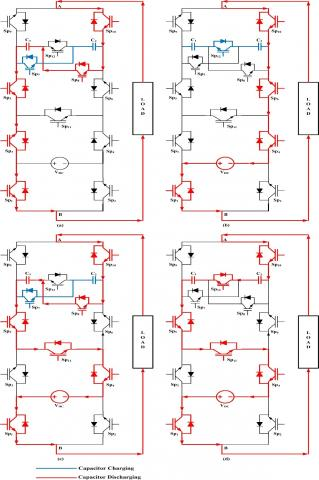Figure 4. Switching modes for negative output voltages (a) $\frac{-V_{D C}}{2}(\mathrm{b})-1 V_{D C}(\mathrm{c}) \frac{-3 V_{D C}}{2}(\mathrm{d})-2 V_{D C}$

Table 1. Switching scheme and positions of Switches, Diodes, and Capacitors

 Timing instants Switching Condition Conducting Diodes Conducting IGBT Switches Position of Capacitors Voltage Level C1 C2 0 - ta VC4 Vc1 ---- Sp2, Sp3, Sp6, Sp9, Sp11, Sp12 ↓ ↓ $+2 V_{D C}$ tf - ta* VC5
3. Phase Disposition Carrier Based PWM

The Phase Disposition Carrier-based Pulse Width Modulation (PDCPWM) control scheme is used for the presented topology shown in Figure 5. The sinusoidal reference signal Vref is comparing with the eight triangular carrier signals Vc1- Vc8  to produce the gate pulses to the switches of the nine-level inverter. The eight carrier signals have the same amplitude AC, frequency fC and phase is arranged one by one based on their amplitudes. For high levels of output, the switches Sp11 & Sp12 are conducting at $V_{r e f}>V_{C 1}$. The switches Sp7 & Sp8 will be turned ON, when the switch Sp12 is turned OFF i.e., at $V_{C 2}<V_{r e f}<V_{C 1}, \quad V_{C 4}<V_{r e f}<V_{C 3}$, $V_{C 6}<V_{r e f}<V_{C 5}, V_{C 8}<V_{r e f}<V_{C 7}$. The switching scheme and states of the capacitors are given in Table 1.

## 5.jpg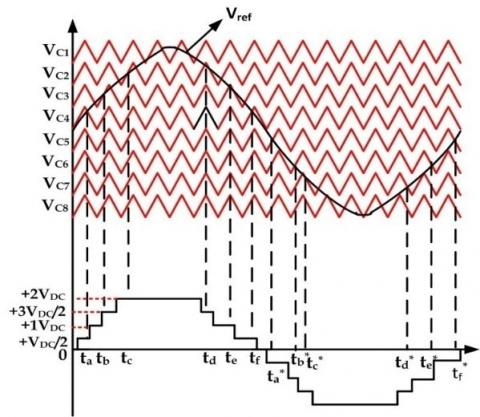Figure 5. Phase Disposition Carrier-based Pulse Width Modulation (PDCPWM) control scheme

From Figure 5, the carrier signals $V_{C 1}-V_{C 4}$ is compared with the positive half cycle of reference sinusoidal signal to produce positive voltage levels. Similarly, the carrier signals $V_{C 5}-V_{C 8}$ are compared with the negative half cycle of reference sinusoidal signal to obtain the negative voltage levels. The positive voltage levels are obtained at time instants $t_{a}-t_{d}$ and are given as

$t_{a}=\frac{\sin ^{-1}\left(\frac{A_{C 4}}{A_{r e f}}\right)}{2 \pi f_{r}}$    (3)

$t_{b}=\frac{\sin ^{-1}\left(\frac{A_{C 3}}{A_{r e f}}\right)}{2 \pi f_{r}}$      (4)

$t_{c}=\frac{\sin ^{-1}\left(\frac{A_{C 2}}{A_{r e f}}\right)}{2 \pi f_{r}}$     (5)

$t_{d}=\pi-\frac{\sin ^{-1}\left(\frac{A_{C 2}}{A_{r e f}}\right)}{2 \pi f_{r}}$     (6)

When $f_{r}=50 \mathrm{Hz} ; \mathrm{A}_{\text {ref}}=3.8$ the time instants of the output voltage are calculated. When the timing instant $t_{a}-t_{b}$  the two capacitors are charging up to the nominal voltage of $\frac{V_{D C}}{2}$. At timing instant $t_{c}-t_{d}$ the two capacitors are discharging and the maximum discharging of the capacitor  is given as:

$\Delta Q_{C i}=\int_{t_{C}}^{t_{d}} i_{L} \sin \left(\omega_{r} t\right) d t$     (7)

where, $t_{c}, t_{d}$  are charging and discharging time instants; i = number of capacitors; $\omega_{r}=2 \pi f_{r}$.

A ripple factor kr= 0.1, the rating of the capacitor is given as:

$C_{i}=\frac{\Delta Q_{C i}}{k_{r}\left(\frac{V_{D C}}{2}\right)}$       (8)

4. Calculation of Losses

The calculation of power loss for a switched capacitor based Multilevel Inverter depends on the Conduction Loss and Switching Loss.

4.1 Conduction loss (PCL)

The reason for occurring the conduction losses are ON state switching resistance RSW,ON, ON state diode resistance RD,ON, and internal resistance of the capacitor RC. At time 0<t < ta, the voltage level is changing from 0 to $\frac{+V_{D C}}{2}$ with three switches, two diodes at zero level and three switches, two diodes, and one capacitor at $\frac{+V_{D C}}{2}$ level. The equivalent resistances of ON state switches, diodes, and capacitors at different voltage levels are given in Table 2.

Table 2. Equivalent resistance of diodes, switches, and capacitors

 Voltage Levels Number of ON State devices Equivalent Resistances Switches Diodes Capacitors 0 3 2 - $3 R_{s w} o_{N}+2 R_{D, O N}$ $\frac{+V_{D C}}{2}$ 3 2 1 $3 R_{s w, O N}+2 R_{D, O N}+R_{C}$ $+1 V_{D C}$ 3 1 - $3 R_{s w, O N}+R_{D, O N}$ $\frac{+3 V_{D C}}{2}$ 5 1 1 $5 R_{s w, O N}+R_{D, O N}+R_{C}$ $+2 V_{D C}$ 6 - 2 $6 R_{s w, O N}+2 R_{C}$

The conduction loss at 0 < t < ta  is given as:

${{P}_{CL1}}={{P}_{0\And \frac{{{V}_{DC}}}{2}}}={{\int\limits_{0}^{ta}{[{{I}_{Load,RMS}}]}}^{2}}\left\lceil [3{{\text{R}}_{sw,ON}}+2{{R}_{D,ON}}+{{R}_{C}}][\frac{{{A}_{ref}}\sin {{\omega }_{r}}t}{{{A}_{C}}}]+[3{{R}_{sw,ON}}+2{{R}_{D,ON}}][1-\frac{{{A}_{ref}}\sin {{\omega }_{r}}t}{{{A}_{c}}}] \right\rceil dt$

where $\omega_{r}=2 \pi f_{r}$;

$I_{L o a d, R M S}=I_{L} \sin \omega_{r} t$     (9)

Similarly, the conduction loss at time instants $t_{a}<\mathrm{t}<t_{b}, t_{b}<\mathrm{t}<t_{c}, t_{c}<\mathrm{t}<t_{d}$  are calculated as follows.

${{P}_{CL2}}={{P}_{\frac{{{V}_{DC}}}{2}\And {{V}_{DC}}}}={{\int\limits_{{{t}_{a}}}^{tb}{[{{I}_{Load,RMS}}]}}^{2}}\left\lceil [3{{\text{R}}_{sw,ON}}+{{R}_{D,ON}}][\frac{{{A}_{ref}}\sin {{\omega }_{r}}t-{{A}_{c}}}{{{A}_{C}}}]+[3{{R}_{sw,ON}}+2{{R}_{D,ON}}+{{R}_{c}}][1-\frac{{{A}_{ref}}\sin {{\omega }_{r}}t-{{A}_{C}}}{{{A}_{c}}}] \right\rceil dt$   (10)

${{P}_{CL3}}={{P}_{{{V}_{DC}}\And \frac{3{{V}_{DC}}}{2}}}={{\int\limits_{{{t}_{a}}}^{{{t}_{\text{c}}}}{[{{I}_{Load,RMS}}]}}^{2}}\left\lceil [5{{\text{R}}_{sw,ON}}+{{R}_{D,ON}}+{{R}_{c}}][\frac{{{A}_{ref}}\sin {{\omega }_{r}}t-2{{A}_{c}}}{{{A}_{C}}}]+[3{{R}_{sw,ON}}+{{R}_{D,ON}}][1-\frac{{{A}_{ref}}\sin {{\omega }_{r}}t-2{{A}_{C}}}{{{A}_{c}}}] \right\rceil dt$        (11)

${{P}_{CL4}}={{P}_{\frac{3{{V}_{DC}}}{2}\And 2{{V}_{DC}}}}={{\int\limits_{{{t}_{c}}}^{{{t}_{d}}}{[{{I}_{Load,RMS}}]}}^{2}}\left\lceil [6{{\text{R}}_{sw,ON}}+2{{R}_{c}}][\frac{{{A}_{ref}}\sin {{\omega }_{r}}t-3{{A}_{c}}}{{{A}_{C}}}]+[5{{R}_{sw,ON}}+{{R}_{D,ON}}+{{R}_{C}}][1-\frac{{{A}_{ref}}\sin {{\omega }_{r}}t-3{{A}_{C}}}{{{A}_{c}}}] \right\rceil dt$        (12)

The output voltage has quarter-wave symmetry, therefore, the total conduction loss is given as:

$P_{C L}=4 P_{C L 1}+4 P_{C L 2}+4 P_{C L 3}+2 P_{C L 4}$       (13)

4.2 Switching loss (PSwL)

The main source of occurring the power losses is switching losses which are occurred due to the switching actions of the semiconductor devices. The switches Sp1, Sp2, Sp7, Sp8, Sp9, Sp10, Sp11, & Sp12 are operated at the low switching frequency $\left(\frac{f_{s w}}{2}\right)$ and Sp3, Sp4, Sp5, Sp6 switches are operated at the high switching frequency $f_{S W}$. At ON-state of the switches, the power loss is given as:

$P_{s w, o n}=f_{s w} \int_{0}^{t_{o n}} V_{s}(t) I_{s}(t) d t=\frac{1}{6} f_{s w} V_{s} I_{s, o n} t_{o n}$    (14)

At OFF-state, the power loss is given as:

$\begin{array}{rl}P_{s w, o f f}=f_{s w} \int_{0}^{t_{o f f}} & V_{s}(t) I_{s}(t) d t \\ & =\frac{1}{6} f_{s w} V_{s} I_{s, o f f} t_{o f f}\end{array}$      (15)

Therefore, the total switching losses is given as:

$P_{s w l}=\sum_{i=1}^{N_{s w}}\left(P_{s w i, o n}+P_{s w i, o f f}\right)$    (16)

where, $N_{s w}$ is a number of switches

5. Results and Discussion

The self-balanced switched-capacitor based nine-level inverter is simulated in MATLAB/SIMULINK software. The switching pulses to the inverter are obtained by comparing the carrier signals of switching frequency $f_{s}=5 K H z$ with the sinusoidal reference signal of frequency $f_{r}=50 \mathrm{Hz}$ is given in Figure 6.

At load, R = 50Ω and L = 50mH, the input current is passed through the inverter and produces the output voltage of 400V and load current of 7.3A from the input DC voltage of 200V is shown in Figure 7 and Figure 8.

A ripple factor of 10%, the voltage across the capacitors for the values of C1 = C2 = 2100µF is given in Figure 9. The capacitors are charged to $\frac{V_{D C}}{2}$ i.e 100V and discharged by the self-balanced process.

## 6.jpg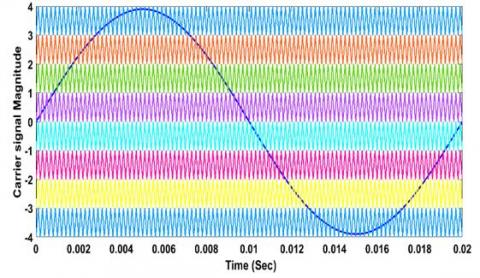Figure 6. Phase disposition carrier based PWM control scheme

## 7.jpgFigure 7. Load voltage of the inverter

## 8.jpgFigure 8. Load current of the inverter

The blocking voltages of the semiconductor switches employed in the inverter given in Figure 10. It shows that the switches SP1, Sp2 (also SP3, Sp4, Sp5, Sp6, Sp9, Sp10, Sp11) must hold up the voltage which is equal to input DC voltage of 200V, and other switches Sp7, Sp8, Sp12 have the blocking voltages of half of input DC voltage i.e. 100V.

At modulation index 0.9, the switched capacitor based nine-level inverter have the Total Harmonic Distortion(THD) is 19.20% at switching frequency $f_{s w}=5 K H z$ is given in Figure 11.

## 9.jpgFigure 9. Capacitor voltages Vc1 and Vc2

## 10.png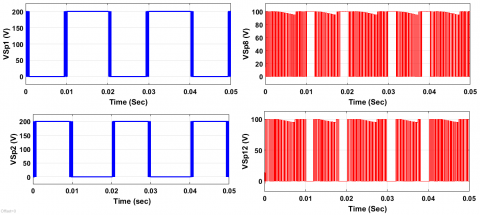Figure 10. Blocking voltages of switches Sp1, Sp2, Sp8, Sp12

## 11.jpgFigure 11. THD analysis at Modulation Index 0.9

6. Comparative Analysis

The comparison analysis between previous topologies and present topology in terms of semiconductor switches, capacitors, and input sources is given in Table 3. From the observation of the table, the topology  used the less number of switches and DC sources. But it has an H-bridge configuration which leads to high PIV and TSV. The four DC sources are connected alternatively in topology  which leads to system expensive and required high ratings of semiconductor switches. In the topology , there is no H-bridge configuration, but it requires a high number of semiconductor switches leads to high TSV and increases the system cost.

Table 3. Comparison of present topology with existing topologies

 Elements Ref.  Ref.  Ref.  Present Topology Swicthes 13 10 19 12 Capacitors 3 0 3 2 DC Sources 1 4 1 1 H-bridges Yes No No No Capacitor Control No No Self-balancing Self-balancing THD 16.40 26.89 28.07 19.20 PIV $6 * 1 V_{D C}$ $3 * 2 V_{D C}$ $4 * 4 V_{D C}$ $2 * 1 V_{D C}$ $4 * 2 V_{D C}$ $4 * 3 V_{D C}$ $19 * 1 V_{D C}$ $9 * V_{d c}$ $3 * \frac{V_{D C}}{2}$ TSV $^{\prime} 28 V_{D C}$ $22 V_{D C}$E $19 V_{D C}$ $9 V_{d c}+\frac{3 V_{D C}}{2}$

In spite of all these topologies, the present topology has many advantages such as it requires only one DC source, two capacitors, twelve semiconductor switches, and no H-bridge configuration. The present nine-level inverter is operating with low PIV i.e., nine switches are operating with $1 V_{D C}$ and three switches are operating with $\frac{V_{D C}}{2}$. Therefore, the blocking voltage across the switches is low and required low rating semiconductor switches which lead to implementation cost is low compared to others.

7. Conclusion

The improved self-balancing switched-capacitor based step up nine-level inverter is simulated in this article. The operating modes of the inverter, charging and discharging states of the capacitors is discussed in detail. The step-up output AC voltage is obtained from the low input DC voltage without using boost converter, transformers. The Phase Disposition Carrier-based PWM (PDCPWM) control scheme is involved for the generation of switching pulses to the inverter. The ratings of the capacitors, switching and conduction losses are obtained mathematically. The comparative analysis between the existing topologies and presented topology depicts the requirement of semiconductor switches is less, operating the switches with low PIV and TSV which leads to cost-effective and improves the performance of the inverter.

Acknowledgment

The authors are very thankful for the support from the management of Vignan’s Foundation for Science, Technology & Research for the successful completion of work.

References

 Vijeh, M., Rezanejad, M., Samadaei, E., Bertilsson, K. (2019). A general review of multilevel inverters based on main submodules: Structural point of view. IEEETransactions on Power Electronics, 34(10): 9479-9502. https://doi.org/10.1109/TPEL.2018.2890649

 Zhang, J.Z., Xu, S., Din, Z., Hu, X. (2019). Hybrid multilevel converters: Topologies, evolutions and verifications. Energies, 12(4): 615. https://doi.org/10.3390/en12040615

 Chen, W.Q., Hotchkiss, E., Bazzi, A. (2018). Reconfiguration of NPC multilevel inverter to mitigate short circuit faults using back to back switches. CPSS Transactions on Power Electronics & Applications, 3(1): 46-55. https://doi.org/10.24295/CPSSTPEA.2018.00005

 McNeill, N., Yuan, X.B., Anthony, P. (2015). High-efficiency NPC multilevel converter using super-junction MOSFETs. IEEE Transactions On Industrial Electronics, 63(1): 25-37. https://doi.org/10.1109/TIE.2015.2466553

 Lei, Y.T., Barth, C., Qin, S.B. Liu, W.C., Moon, I., Stillwell, A., Chou, D., Foulkes, T., Ye, Z.C., Liao, Z.T., Pilawa-Podgurski, R.C.N. (2017). A 2-kW single-phase seven-level flying capacitor multilevel inverter with an active energy buffer. IEEE Transactions On Power Electronics, 32(11): 8570–8581. https://doi.org/10.1109/TPEL.2017.2650140

 Stillwell, A., Podgurski, R.C. (2019). A five level flying capacitor multilevel converter with integrated auxilliary power supply and start-up. IEEE Transaction on Power Electronics, 34(3): 2900-2913. https://doi.org/10.1109/TPEL.2018.2843777

 Ye, M.Y., Ren, W., Chen, L., Wei, Q.W., Song, G.Z., Li, S. (2019). Research on power-balance control strategy of CHB multilevel inverter based on TPWM. IEEE Access, 7: 157226-157240. https://doi.org/10.1109/ACCESS.2019.2950064

 Lee, S.S., Sidorov, M., Idris, N.R.N., Heng, Y.N. (2018). A symmetrical cascaded compact-module multilevel inverter (CCM-MLI) with pulsewidth modulation. IEEE Transactions On Industrial Electronics, 65(6): 4631-4639. https://doi.org/10.1109/TIE.2017.2772209

 Gupta, K.K., Ranjan, A., Bhatnagar, P., Sahu, L.K., Jain, S. (2016). Multilevel inverter topologies with reduced device count: A review. IEEE Transactions On Power Electronics, 31(1): 135–151. https://doi.org/10.1109/TPEL.2015.2405012

 Saeedian, M., Pouresmaeil, E., Samadaei, E., Rodrigues, E.M.G., Godina, R., Marzband, M. (2019). An innovative dual-boost nine-level inverter with low-voltage rating switches. Energies, 12(2): 207. https://doi.org/10.3390/en12020207

 Akpınar, E., Balıkcı, A., Durbaba, E., Azizo, B.T. (2019). Single-phase transformerless photovoltaic inverter with suppressing resonance in improved H6. IEEE Transactions On Power Electronics, 4(9): 8304–8316. https://doi.org/10.1109/TPEL.2018.2886054

 Roy, J., Xia, Y.L., Ayyanar, R. (2019). High step-up transformerless inverter for AC module applications with active power decoupling. IEEE Transactions On Industrial Electronics, 66(5). https://doi.org/10.1109/TIE.2018.2860538

 Wang, L., Wu, Q.H., Tang, W.H. (2017). Novel cascaded switched-diode multilevel inverter for renewable energy integration. IEEE Transactions On Energy Conversion, 32(4): 1574-1582. https://doi.org/10.1109/TEC.2017.2710352

 Hinago, Y., Koizumi, H. (2012). A switched-capacitor inverter using series/parallel conversion with inductive load. IEEE Transactions On Industrial Electronics, 59(2): 878–887. https://doi.org/10.1109/TIE.2011.2158768

 Gupta, K.K., Jain, S. (2014). A novel multilevel inverter based on switched DC sources. IEEE Transactions On Industrial Electronics, 61(7): 3269-3278. https://doi.org/10.1109/TIE.2013.2282606

 Vahedi, H., Labbé, P.A., Al-Haddad, K. (2016). Sensor-less five-level packed U-Cell (PUC5) inverter operating in stand-alone and grid-connected modes. IEEE Transactions On Industrial Informatics, 12(1): 361-370. https://doi.org/10.1109/TII.2015.2491260

 Nakagawa, Y., Koizumi, H. (2018). A boost type nine-level switched capacitor inverter. IEEE Transactions on Power Electronics, 34(7): 6522-6532. https://doi.org/10.1109/TPEL.2018.2876158

 Taghvaie, A., Adabi, J., Rezanejad, M. (2018). A self-balanced step-up multilevel inverter based on switched-capacitor structure. IEEE Transactions On Power Electronics, 33(1): 199-209. https://doi.org/10.1109/TPEL.2017.2669377

 Basha, S.G., Venkatesan, M. (2019). Simulation of self-balanced based step-up switched capacitor nine level inverter with MCPWM scheme. International Journal on Emerging Technologies, 10(3): 87-95.